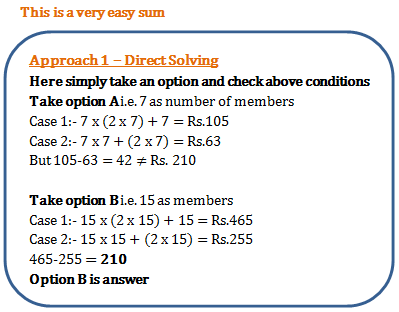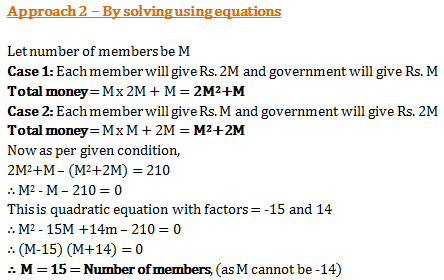# Problems on Numbers - Quantitative Aptitude (MCQ) questions for Q. 29269

Q.  A group wanted to renovate their club. Each member contributed an amount equal to twice the number of members in the club. But the government contributed same amount as the number of members. If each member had contributed the same amount as the number of members and the government had given an amount twice the number of members, then they would have Rs. 210 less. How many members are there?
- Published on 11 Apr 17

a. 7
b. 15
c. 14
d. 24